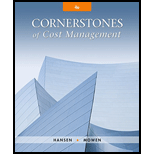# Lilly Company is planning to buy a set of special tools for its grinding operation. The cost of the tools is $18,000. The tools have a three-year life and qualify for the use of the three-year MACRS. The tax rate is 40 percent; the cost of capital is 12 percent. Required: 1. Calculate the present value of the tax depreciation shield, assuming that straight-line depreciation with a half-year life is used. 2. Calculate the present value of the tax depreciation shield, assuming that MACRS is used. 3. What is the benefit to the company of using MACRS?BuyFindarrow_forward ### Cornerstones of Cost Management (C... 4th Edition Don R. Hansen + 1 other Publisher: Cengage Learning ISBN: 9781305970663 #### Solutions Chapter SectionBuyFindarrow_forward ### Cornerstones of Cost Management (C... 4th Edition Don R. Hansen + 1 other Publisher: Cengage Learning ISBN: 9781305970663 Chapter 19, Problem 18E Textbook Problem 5 views ## Lilly Company is planning to buy a set of special tools for its grinding operation. The cost of the tools is$18,000. The tools have a three-year life and qualify for the use of the three-year MACRS. The tax rate is 40 percent; the cost of capital is 12 percent.Required: 1. Calculate the present value of the tax depreciation shield, assuming that straight-line depreciation with a half-year life is used. 2. Calculate the present value of the tax depreciation shield, assuming that MACRS is used. 3. What is the benefit to the company of using MACRS?

1.

To determine

Calculate the present value of the after tax depreciation, assume that straight-line depreciation with a half-year life is used.

### Explanation of Solution

Net present value method (NVP): Net present value method is the method which is used to compare the initial cash outflow of investment with the present value of its cash inflows. In the net present value, the interest rate is desired by the business based on the net income from the investment, and it is also called as the discounted cash flow method.

Calculate the present value of the after tax depreciation, assume that straight-line depreciation with a half-year life is used:

 Year Annual deprecation (A) (1) Deprecation after tax (B)(A×40%) Discount factor @ 12% (C) Present value (B×C) 1 3,000 $1,200 0.893$1,072 2 6,000 $2,400 0.797$1,913 3 6,000 \$2,400 0

2.

To determine

Calculate the present value of the after tax depreciation, assume that MACRS method is used.

3.

To determine

State the benefits that the company enjoys when using MACRS method of depreciation.

### Still sussing out bartleby?

Check out a sample textbook solution.

See a sample solution

#### The Solution to Your Study Problems

Bartleby provides explanations to thousands of textbook problems written by our experts, many with advanced degrees!

Get Started

Find more solutions based on key concepts
Which inventory method always follows the actual physical flow of merchandise?

College Accounting, Chapters 1-27 (New in Accounting from Heintz and Parry)

Why do economists oppose policies that restrict trade among nations?

Principles of Microeconomics (MindTap Course List)

PRICE AND YIELD An 8% semiannual coupon bond matures in 5 years. The bond has a face value of 1,000 and a curre...

Fundamentals of Financial Management, Concise Edition (with Thomson ONE - Business School Edition, 1 term (6 months) Printed Access Card) (MindTap Course List)

What are the steps in entering international markets?

Foundations of Business (MindTap Course List)

Why does a bank keep a signature card on file for customers accounts?

College Accounting (Book Only): A Career Approach